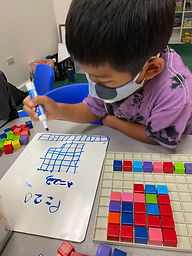## Ms. Brianne

### Target 1​

###### Lesson Type:

Continuation

Measurment

:

Techniques and Formulas

Find the perimeter of a given shape.

###### 1:

Understand that perimeter is the distance around a figure.

###### 2:

Understand that perimeter can be found by adding the lengths of the sides of a figure.

###### 3:

Understand that figures with the same perimeter can look different.

3rd

###### Vocabulary:

Perimeter, Length, Width, Area

Activities:

• Students understood that perimeter is the distance around a 2D figure and area measures its surface inside.
• Students built different figures with the same perimeter, and then figured out each one's area. They noticed that the area of 2 figures can be different even though the perimeter was the same.
• Students found the perimeter and area for the different pentominoes. They noticed that even though the pentominoes looked different, they all had a perimeter of 12 and an area of 5, except for one piece.### Home Exploration

###### Guiding Questions:## Absent Students:

### Target 2

:

###### 1:

Understand that a tessellation is a repeated geometric figure that covers a plane without gaps or overlaps.

###### 2:

Construct a tessellation and use it to create a pattern in which there are no empty spaces.

4th

###### Vocabulary:

Tessellation, Pattern, Repeat, Space

Activities:

• Students used a square post-it to create their own geometric figure.
• Students used their figure to create a tessellation that filled up their entire paper with no gaps in between.### Home Exploration

###### Guiding Questions:### Target 3

:

###### Vocabulary:

Activities:### Home Exploration